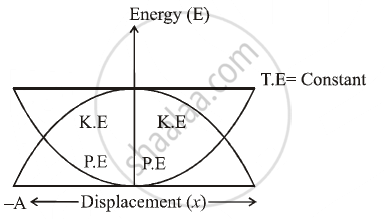# State an Expression for K. E. (Kinetic Energy) and P. E. (Potential Energy) at Displacement ‘X’ for a Particle Performing Linear S.H. M. Represent Them Graphically. - Physics

Short Note

State an expression for K. E. (kinetic energy) and P. E. (potential energy) at displacement ‘x’ for a particle performing linear S.H. M. Represent them graphically. Find the displacement at which K. E. is equal to P. E.

#### Solution

:.K.E.=1/2mw^2(A^2-X^2)

P.E.=1/2mw^2X^2K.E. = P.E.

1/2K(A^2-X^2)=1/2KX^2

∴ A2=2X2

:.X^2=A^2/2

:.X=A/sqrt2

Concept: K.E.(Kinetic Energy) and P.E.(Potential Energy) in S.H.M.
Is there an error in this question or solution?
2013-2014 (March)

Share